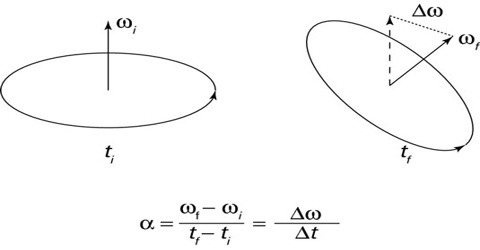# Angular Acceleration

Angular acceleration

Angular acceleration is the rate of change of angular Velocity with respect to time. In many cases angular velocity of a rotating particle increases or decreases. If angular velocity changes then it is understood that the particle is moving with angular acceleration. It is a vector quantity, consisting of a magnitude component and either of two defined directions or senses.

Average angular acceleration of a rotating particle means rate of change of angular velocity for a fixed time interval.

So, if for a very small time interval ∆t the change of angular velocity is ∆ω, then average angular acceleration during that tune interval is,

α = ∆ω/∆t

If time interval tends to zero, thus we get instantaneous angular acceleration.By applying the rule of calculus, we get,

α = lit∆t→0 ∆ω/∆t;

or, α = dω/dt = d/dt (dθ/dt) = d2θ/dt2

Angular acceleration means instantaneous angular acceleration.